Courses

# Cache Memory - Practice Quiz - 1

## 20 Questions MCQ Test RRB JE for Computer Science Engineering | Cache Memory - Practice Quiz - 1

Description
This mock test of Cache Memory - Practice Quiz - 1 for Computer Science Engineering (CSE) helps you for every Computer Science Engineering (CSE) entrance exam. This contains 20 Multiple Choice Questions for Computer Science Engineering (CSE) Cache Memory - Practice Quiz - 1 (mcq) to study with solutions a complete question bank. The solved questions answers in this Cache Memory - Practice Quiz - 1 quiz give you a good mix of easy questions and tough questions. Computer Science Engineering (CSE) students definitely take this Cache Memory - Practice Quiz - 1 exercise for a better result in the exam. You can find other Cache Memory - Practice Quiz - 1 extra questions, long questions & short questions for Computer Science Engineering (CSE) on EduRev as well by searching above.
QUESTION: 1

### A file system uses an in-memory cache to cache disk blocks. The miss rate of the cache is shown in the figure. The latency to read a block from the cache is ms and to read a block from the disk is ms. Assume that the cost of checking whether a block exists in the cache is negligible. Available cache sizes are in multiples of MB.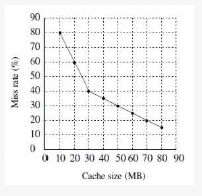The smallest cache size required to ensure an average read latency of less than ms is _________ MB.

Solution:

For 20 MB, the miss rate is 60% and for 30 MB, it is 40%. Thus, the smallest cache size required to ensure an average read latency of less than 6 ms is 30 MB.

QUESTION: 2

Solution:
QUESTION: 3

### The access times of the main memory and the Cache memory, in a computer system, are 500 n sec and 50 n sec, respectively. It is estimated that 80% of the main memory request are for read the rest for write. The hit ratio for the read access only is 0.9 and a write-through policy (where both main and cache memories are updated simultaneously) is used. Determine the average time of the main memory.

Solution:
QUESTION: 4

The principle of locality justifies the use of

Solution:

It is D.
Locality of reference is actually the frequent accessing of any storage location or some value. We can say in simple language that whatever things are used more frequently, they are stored in the locality of reference. So we have cache memory for the purpose.

QUESTION: 5

A computer system has a 4 K word cache organized in block-set-associative manner with 4 blocks per set, 64 words perblock. The number of bits in the SET and WORD fields of the main memory address format is:

Solution:

Number of sets = 4K /(64*4) = 16. So, we need 4 bits to identify a set => SET = 4 bits.
64 words per block means WORD is 6 bits.
So, (D)

QUESTION: 6

A computer system has a three level memory hierarchy, with access time and hit ratios as shown below: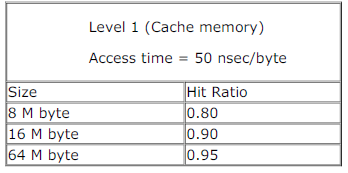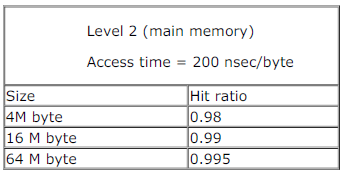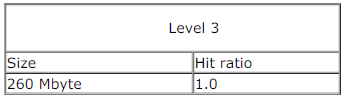A. What should be the minimum sizes of level 1 and 2 memories to achieve an average access time of less than 100 nsec
B. What is the average access time achieved using the chosen sizes of level 1 and level 2 memories?

Solution: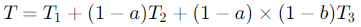Here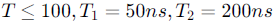and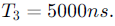On substituting the a,b for the first case we get

T = 95ns for a = 0.8 and b = 0.995. i.e., L1 = 8M and L2 = 64M.

T = 75ns for a = 0.9 and b = 0.99. i.e., L1 = 16M and L2 = 4M

b.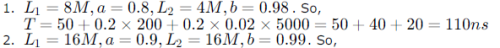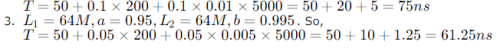QUESTION: 7

For a set-associative Cache organization, the parameters are as follows: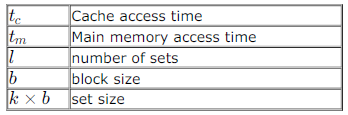Calculate the hit ratio for a loop executed 100 times where the size of the loop is n x b and n = k x m is a non-zero integer and 1 < m ≤ l.
Give the value of the hit ratio for l = 1

Solution:

Size of the loop = n × b = k × m × b

Size of a set = k × b (k − way associative)

Here, size of the loop is smaller than size of a set as . Now, however be the mapping (whether all be mapped to the same set or not), we are guaranteed that the entire loop is in cache without any replacement.
For the first iteration:

No. of accesses = n × b

No. of misses = n  as each new block access is a miss and loop body has blocks each of size for a total size of .
For, the remaining 99 iterations:

No. of accesses = n × b

No. of misses = 0

So, total no. of accesses = 100nb
Total no. of hits = Total no. of accesses − Total no. of misses
= 100nb − n
So, hit ratio =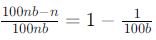The hit ratio is independent if l, so for l = 1 also we have hit ratio = 1-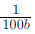QUESTION: 8

The main memory of a computer has 2 cm blocks while the cache has 2c blocks. If the cache uses the set associativemapping scheme with 2 blocks per set, then block k of the main memory maps to the set

Solution:

Number of cache blocks = 2c
Number of sets in cache = 2c/2 = c since each set has 2 blocks. Now, a block of main memory gets mapped to a set (associativity of 2 just means there are space for 2 memory blocks in a cache set), and we have 2cm blocks being mapped to c sets. So, in each set 2m different main memory blocks can come and block k of main memory will be mapped to k
mod c.

QUESTION: 9

More than one word are put in one cache block to

Solution:

exploit the spatial locality of reference in a program as, if the next locality is addressed immediately, it will already be in the cache.
Consider the scenario similar to cooking, where when an ingredient is taken from cupboard, you also take the near by ingredients along with it- hoping that they will be needed in near future.

QUESTION: 10

A CPU has 32-bit memory address and a 256 KB cache memory. The cache is organized as a 4-way set associative cache with cache block size of 16 bytes.
a. What is the number of sets in the cache?
b. What is the size (in bits) of the tag field per cache block?
c. What is the number and size of comparators required for tag matching?
d. How many address bits are required to find the byte offset within a cache block?
e. What is the total amount of extra memory (in bytes) required for the tag bits?

Solution:

What is the number of sets in the cache?
Number of sets = Cache memory/(set associativity * cache block size)
= 256KB/(4*16 B)
= 4096
What is the size (in bits) of the tag field per cache block?
Number of bits required to identify a particular set = 12 (Number of sets = 4096)
Number of bits required to identify a paticular location in cache line = 4 (cache block size = 16)
size of tag field = 32 -12 -4 = 16-bit
What is the number and size of comparators required for tag matching?

We use 4-way set associate cache. So, we need 4 comparators each of size 16 bits

How many address bits are required to find the byte offset within a cache block?
Cache block size is 16 byte. so 4 bits are required to find the byte offset within a cache block.
What is the total amount of extra memory (in bytes) required for the tag bits?
size of tag = 16 bits
Number of sets = 4096
Set associativity = 4
Extramemory required to store the tag bits = 16 * 4096 * 4 bits = 218 bytes

QUESTION: 11

In a C program, an array is declared as float A. Each array element is 4 Bytes in size, and the starting address of the array is 0x00000000. This program is run on a computer that has a direct mapped data cache of size 8 Kbytes, with block (line) size of 16 Bytes.
a. Which elements of the array conflict with element A in the data cache? Justify your answer briefly.
b. If the program accesses the elements of this array one by one in reverse order i.e., starting with the last element and ending with the first element, how many data cache misses would occur? Justify your answer briefly. Assume that the data cache is initially empty and that no other data or instruction accesses are to be considered.

Solution:

(a)
Data cache size = 8KB.
Block line size = 16B.
Since each array element occupies 4B, four consecutive array elements occupy a block line (elements are aligned as
Number of cache blocks = 8KB/16B = 512. Number of cache blocks needed for the array = 2048/4 = 512. So, all the array
elements has its own cache block and there is no collision.
We can also explain this with respect to array address. Starting address is 0x00000000 = 0b0000..0 (32 0's). Ending
address is 0x00001FFF = 0b0000..0111111111111 (4*2048 = 8192 location).
Here, the last 4 bits are used as OFFSET bits and the next 9 bits are used as SET bits. So, since the ending address is not
extending beyond these 9 bits, all cache accesses are to diff sets.
(b) If the last element is accessed first, its cache block is fetched. (which should contain the previous 3 elements of the
array also since each cache block hold 4 elements of array and 2048 is and exact multiple of 4). Thus, for every 4
accesses, we will have a cache miss => for 2048 accesses we will have 512 cache misses. (This would be same even if we
access array in forward order).

QUESTION: 12

Consider a small two-way set-associative cache memory, consisting of four blocks. For choosing the block to be replaced,use the least recently used (LRU) scheme. The number of cache misses for the following sequence of block addresses is8, 12, 0, 12, 8

Solution:

We have 4 blocks and 2 blocks in a set => there are 2 sets. So blocks will go to sets as follows: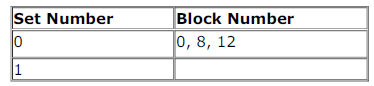since the lowest bit of block address is used for indexing into the set.
So, 8, 12 and 0 first miss in cache with 0 replacing 8 (there are two slots in each set due to 2-way set) and then 12 hits in
cache and 8 again misses. So totally 4 misses.

QUESTION: 13

Consider a system with 2 level cache. Access times of Level 1 cache, Level 2 cache and main memory are 1 ns, 10 ns, and500 ns, respectively. The hit rates of Level 1 and Level 2 caches are 0.8 and 0.9, respectively. What is the average accesstime of the system ignoring the search time within the cache?

Solution:

By default we consider hierarchical access - because that is the common implementation and simultaneous access cache has great practical difficulty. But here the question is a bit ambiguous -- it says to ignore search time within the cache - usually search is applicable for an associative cache but here no such information given. So, may be they are telling to
ignore the search time for L1 and just consider the time for L2 for an L1 miss and similarly just consider memory access time for L2 miss. This is nothing but simultaneous access.
Access time for hierarchical access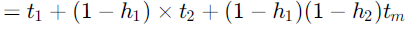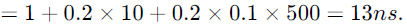Access time for simultaneous access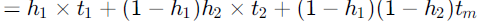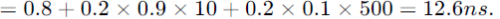Both options in choice - :O

QUESTION: 14

Consider a fully associative cache with 8 cache blocks (numbered 0-7) and the following sequence of memory block requests:
4, 3, 25, 8, 19, 6, 25, 8, 16, 35, 45, 22, 8, 3, 16, 25, 7
If LRU replacement policy is used, which cache block will have memory block 7?

Solution:

When 45 comes, the cache contents are 4 3 25 8 19 6 16 35
LRU array (first element being least recently used) [4 3 19 6 25 8 16 35]
So, 45 replaces 4 45 3 25 8 19 6 16 35 [3 19 6 25 8 16 35 45]
Similarly 22 replaces 3 to give
45 22 25 8 19 6 16 35 [19 6 25 8 16 35 45 22]
8 hits in cache 45 22 25 8 19 6 16 35 [19 6 25 16 35 45 22 8]
3 replaces 19 45 22 25 8 3 6 16 35 [6 25 16 35 45 22 8 3]
16 and 25 hits in cache
45 22 25 8 3 6 16 35 [6 35 45 22 8 16 25 3]
Finally 7 replaces 6, which is in block 5.

QUESTION: 15

Consider a direct mapped cache of size 32 KB with block size 32 bytes. The CPU generates 32 bit addresses. The number ofbits needed for cache indexing and the number of tag bits are respectively,

Solution:

Number of blocks = cache size/block size = 32KB/32 = 1024 bytes. So, indexing requires 10 bits. Number of OFFSET bits required to access 32 bit block = 5. So, number of TAG bits = 32 - 10 - 5 = 17. So, answer is (A).

QUESTION: 16

Consider a 2-way set associative cache memory with 4 sets and total 8 cache blocks (0-7) and a main memory with 128 blocks (0-127). What memory blocks will be present in the cache after the following sequence of memory block references if LRU policy is used for cache block replacement. Assuming that initially the cache did not have any memory block from the
current job?
0 5 3 9 7 0 16 55

Solution:

128 main memory blocks are mapped to 4 sets in cache. So, each set maps 32 blocks each. And in each set there is place for two blocks (2-way set).
Now, we have 4 sets meaning 2 index bits. Also, 32 blocks going to one set means 5 tag bits.
Now, these 7 bits identify a memory block and tag bits are placed before index bits. (otherwise adjacent memory references- spatial locality- will hamper cache performance)
So, based on the two index bits (lower 2 bits) blocks will be going to sets as follows: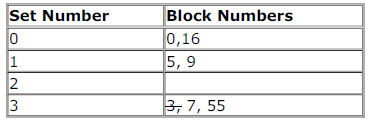Since, each set has only 2 places, 3 will be throw out as its the least recently used block. So, final content of cache will be
0 5 7 9 16 55
(C) choice.

QUESTION: 17

Consider two cache organizations. First one is 32 kb 2-way set associative with 32 byte block size, the second is of same size but direct mapped. The size of an address is 32 bits in both cases . A 2-to-1 multiplexer has latency of 0.6 ns while a k - bit comparator has latency of

k/10 ns The hit latency of the set associative organization is hwhile that of direct mapped is h2
.
The value of h1 is:

Solution:

Cache size is 32 KB and cache block size is 32 B. So,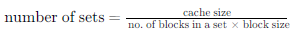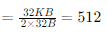So, number of index bits needed = 9 ( since 29 = 512). Number of offset bits = 5 (since 25 = 32 B is the block size and assuming byte addressing). So, number of tag bits = 32 - 9 - 5 = 18 (as memory address is of 32 bits).
So, time for comparing the data = Time to compare the data + Time to select the block in set = 0.6 + 18/10 ns = 2.4 ns.
(Two comparisons of tag bits need to be done for each block in a set, but they can be carried out in parallel and the succeeding one multiplexed as the output).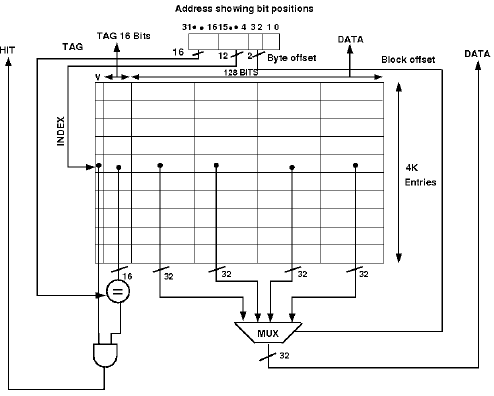QUESTION: 18

Consider two cache organizations. First one is 32 kb 2-way set associative with 32 byte block size, the second is of same size but direct mapped. The size of an address is 32 bits in both cases . A 2-to-1 multiplexer has latency of 0.6 ns while a k - bit comparator has latency of

k/10 ns The hit latency of the set associative organization is hwhile that of direct mapped is h2
.
The value of h1 is:

Solution:

word offset=5bit
block offset=32kb/32=10bit
so tag bit=32-10-5=17bit
hit latency=mux delay+comparator delay
1. mux is not required in direct mapped cache coz we have only one comprator(IF IT IS 2 WAY SET ASSOCITATIVE THEN
COMPRATOR WILL BE 2 AND WE NEED A MUX OF 2-TO-1 TO DECIDE HIT/MISS) so mux delay=0..
2 comp. delay=k/10=17/10=1.7.
so h2 =1.7

QUESTION: 19

A CPU has a 32 KB direct mapped cache with 128 byte-block size. Suppose A is two dimensional array of size with elements that occupy 8-bytes each. Consider the following two C code segments, P1 and P2.
P1:
for (i=0; i<512; i++)
{
for (j=0; j<512; j++)
{
x +=A[i] [j];
}
}
P2:
for (i=0; i<512; i++)
{
for (j=0; j<512; j++)
{
x +=A[j] [i];
}
}
P1 and P2 are executed independently with the same initial state, namely, the array A is not in the cache and i, j, x are in registers. Let the number of cache misses experienced by P1 be M1 and that for P2 be M2.
The value of M1 is:

Solution:

Code being C implies array layout is row-major.
When A is fetched, 128 consecutive bytes are moved to cache. So, for the next 128/8 -1= 15 memory references
there won't be a cache miss. For the next iteration of i loop also the same thing happens as there is no temporal locality in the code. So, number of cache misses for P1
= (512/16) × 512
= 32 × 512
= 214 = 16384

QUESTION: 20

A CPU has a 32 KB direct mapped cache with 128 byte-block size. Suppose A is two dimensional array of size with elements that occupy 8-bytes each. Consider the following two C code segments, P1 and P2.
P1:
for (i=0; i<512; i++)
{
for (j=0; j<512; j++)
{
x +=A[i] [j];
}
}
P2:
for (i=0; i<512; i++)
{
for (j=0; j<512; j++)
{
x +=A[j] [i];
}
}
P1 and P2 are executed independently with the same initial state, namely, the array A is not in the cache and i, j, x are in
registers. Let the number of cache misses experienced by P1 be M1 and that for P2 be M2.
The value of the ratio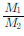Solution:

Number of Cache Lines

In 1 Cache Line

P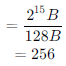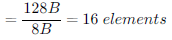P2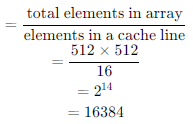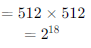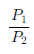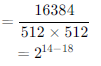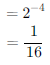It is so because for
P1 for every line there is a miss, and once a miss is processed we get 16 elements in memory. So another miss happens after 16 elements.
for
P2 for every element there is a miss coz storage is row major order(by default) and we are accessing column wise.